#Exact and Nonexact EquationsAll the techniques we have reviewed so far were not of a general nature since in each case the equations themselves were of a special form. So, we may ask, what to do for the general equation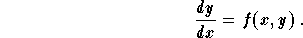Let us first rewrite the equation into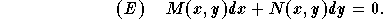This equation will be called exact if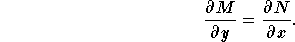,

and nonexact otherwise. The condition of exactness insures the existence of a function F(x,y) such that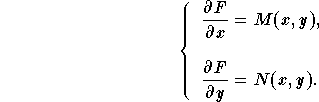When the equation (E) is exact, we solve it using the following steps:

(1)
Check that the equation is indeed exact;
(2)
Write down the system(3)
Integrate either the first equation with respect of the variable x or the second with respect of the variable y. The choice of the equation to be integrated will depend on how easy the calculations are. Let us assume that the first equation was chosen, then we get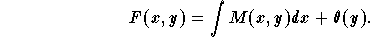The function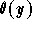should be there, since in our integration, we assumed that the variable y is constant.

(4)
Use the second equation of the system to find the derivative of. Indeed, we have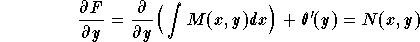,

which implies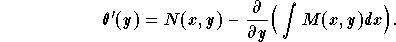Note thatis a function of y only. Therefore, in the expression giving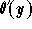the variable, x, should disappear. Otherwise something went wrong!

(5)
Integrate to find;
(6)
Write down the function F(x,y);
(7)
All the solutions are given by the implicit equation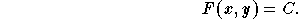(8)
If you are given an IVP, plug in the initial condition to find the constant C.

You may ask, what do we do if the equation is not exact? In this case, one can try to find an integrating factor which makes the given differential equation exact.[Differential Equations] [Algebra] [Trigonometry ]
[Calculus] [Complex Variables] [Matrix Algebra]S.O.S MATHematics home page

Do you need more help? Please post your question on our S.O.S. Mathematics CyberBoard.Author: Mohamed Amine Khamsi
Last Update 6-24-98